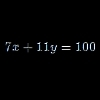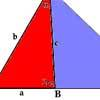Search by Topic

Filter by: Content type:
Age range:
Challenge level:

There are 23 results

Broad Topics > Algebraic expressions, equations and formulae > Diophantine equationsLetter Land

Age 11 to 14 Challenge Level:

If: A + C = A; F x D = F; B - G = G; A + H = E; B / H = G; E - G = F and A-H represent the numbers from 0 to 7 Find the values of A, B, C, D, E, F and H.CD Heaven

Age 14 to 16 Challenge Level:

All CD Heaven stores were given the same number of a popular CD to sell for £24. In their two week sale each store reduces the price of the CD by 25% ... How many CDs did the store sell at. . . .Fibs

Age 11 to 14 Challenge Level:

The well known Fibonacci sequence is 1 ,1, 2, 3, 5, 8, 13, 21.... How many Fibonacci sequences can you find containing the number 196 as one of the terms?Solving with Euclid's Algorithm

Age 14 to 18 Challenge Level:

A java applet that takes you through the steps needed to solve a Diophantine equation of the form Px+Qy=1 using Euclid's algorithm.Euclid's Algorithm II

Age 16 to 18

We continue the discussion given in Euclid's Algorithm I, and here we shall discover when an equation of the form ax+by=c has no solutions, and when it has infinitely many solutions.Euclid's Algorithm I

Age 16 to 18

How can we solve equations like 13x + 29y = 42 or 2x +4y = 13 with the solutions x and y being integers? Read this article to find out.Why Stop at Three by One

Age 16 to 18

Beautiful mathematics. Two 18 year old students gave eight different proofs of one result then generalised it from the 3 by 1 case to the n by 1 case and proved the general result.Are You Kidding

Age 14 to 16 Challenge Level:

If the altitude of an isosceles triangle is 8 units and the perimeter of the triangle is 32 units.... What is the area of the triangle?Deep Roots

Age 14 to 16 Challenge Level:

Find integer solutions to: $\sqrt{a+b\sqrt{x}} + \sqrt{c+d.\sqrt{x}}=1$Plutarch's Boxes

Age 11 to 14 Challenge Level:

According to Plutarch, the Greeks found all the rectangles with integer sides, whose areas are equal to their perimeters. Can you find them? What rectangular boxes, with integer sides, have. . . .In Particular

Age 14 to 16 Challenge Level:

Can you find formulas giving all the solutions to 7x + 11y = 100 where x and y are integers?Whole Numbers Only

Age 11 to 14 Challenge Level:

Can you work out how many of each kind of pencil this student bought?Not a Polite Question

Age 11 to 14 Challenge Level:

When asked how old she was, the teacher replied: My age in years is not prime but odd and when reversed and added to my age you have a perfect square...Coffee

Age 14 to 16 Challenge Level:

To make 11 kilograms of this blend of coffee costs £15 per kilogram. The blend uses more Brazilian, Kenyan and Mocha coffee... How many kilograms of each type of coffee are used?Hallway Borders

Age 11 to 14 Challenge Level:

What are the possible dimensions of a rectangular hallway if the number of tiles around the perimeter is exactly half the total number of tiles?Double Angle Triples

Age 16 to 18 Challenge Level:

Try out this geometry problem involving trigonometry and number theoryOur Ages

Age 14 to 16 Challenge Level:

I am exactly n times my daughter's age. In m years I shall be ... How old am I?Code to Zero

Age 16 to 18 Challenge Level:

Find all 3 digit numbers such that by adding the first digit, the square of the second and the cube of the third you get the original number, for example 1 + 3^2 + 5^3 = 135.Exhaustion

Age 16 to 18 Challenge Level:

Find the positive integer solutions of the equation (1+1/a)(1+1/b)(1+1/c) = 2Age 16 to 18 Challenge Level:

If the last four digits of my phone number are placed in front of the remaining three you get one more than twice my number! What is it?Rudolff's Problem

Age 14 to 16 Challenge Level:

A group of 20 people pay a total of £20 to see an exhibition. The admission price is £3 for men, £2 for women and 50p for children. How many men, women and children are there in the group?Upsetting Pitagoras

Age 14 to 18 Challenge Level:

Find the smallest integer solution to the equation 1/x^2 + 1/y^2 = 1/z^2